## John Shackell and Simon Thompson

### Symbolic asymptotics

Most general-purpose computer algebra systems contain packages for the calculation of limits and other types of asymptotic computation. However these are almost entirely based on power series and L'Hôpital's Rule. For non-linear problems in particular, such methods are often insufficient.

In fact there has been substantial algorithmic development in this area over the past ten years, and modulo some long-standing difficulties with determining the signs of constants, effective methods now exist for determining the asymptotic behaviour for some fairly general classes of functions; these include exp-log functions, Liouvillian functions, inverse and implicit functions.

Symbolic asymptotics can thus be seen as an active area of mathematical research, with impact on the practice of symbolic mathematics as implemented in systems such as Axiom. It also provides an ideal source of case study topics which bridge computer algebra and reasoning; we now look at two links in more detail.

In symbolic asymptotics, expressions of the form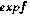have to be treated in different ways according to whether the limit of f is finite or infinite. For example if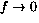, thenneeds to be treated as the infinite series, whereas this is not appropriate if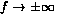. Similarly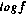and fr, r constant, need special treatment in the cases when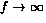and. This was handled from a mathematical viewpoint by Shackell by giving different names to the functions in the different cases, e.g. zexp f when. The use of pre- and post-conditions would seem natural within the proposed project: a pre-condition can be placed upon an argument having a certain asymptotic behaviour, whilst proof can establish post-conditions or invariants also relating to behaviour in the limit.

Very often in symbolic asymptotics, it is important to know whether or not some sub-expression is functionally equivalent to zero. Division is an obvious example, but additionally one often obtains an asymptotic expression for a function f in the form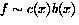, where b(x) is some standard function such as ex and c(x) is given by an expression involving terms which grow less rapidly than b(x). This means that we have asymptotic information about f provided that c(x) is not equivalent to zero. Here again, logical conditions can play a useful role.

### The case study

The case study will involve work in formalising some areas of symbolic asymptotics, including the following topics.

Star Products. These give a new way of expressing asymptotic growth, which might have considerable advantages over the existing ones. Essentially one defines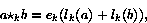where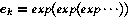(k `exp's) and similarly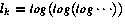. So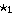is just ordinary multiplication obtained by adding logs and exponentiating. The idea is to regard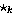as a sort of generalized multiplication and to use these for different k.

We anticipate that there will be a considerable amount of work to be done in reworking existing algorithms to use star-product expressions. This is unlikely to be an entirely routine matter, since experience has shown that it is sometimes substantially harder to obtain multiseries algorithms than ones using nested expansions, and star products generalize both.

Modular Methods in Zero Equivalence. The zero equivalence methods of Shackell suffer from the classic gcd problems of growth in intermediate coefficients and exponents. Work in progress suggests that modular methods may offer some assistance here. Practical and theoretical work need to go hand in hand here, and moreover this topic relates closely with other areas of the project.

Beyond these topics we see possibilities for a student to work on the possibility of extending current work in symbolic asymptotics concerned with implicit functions, oscillating coefficients inverse functions and Pfaffian chains.